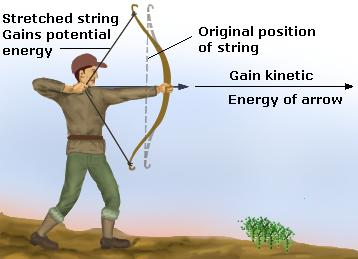Potential energy is associated with the possibility of converting the stored energy of an object at rest to other forms of energy, kinetic energy being the most proximate conversion.

Potential energy is hugely dependent on the position of an object. In fact, potential energy is the system of the energy related to an object’s position. An object’s position when not in motion is concerned with potential energy.Potential energy is a type of force. It is associated with other types of forces such as conservative force, Coulomb force, and intermolecular force. It is said that most forms of energy has a corresponding potential energy.

Various types of energy includes: gravitational potential energy, chemical potential energy, thermal potential energy, electrical potential energy, among others.The potential energy of an object directly involves the object’s location in a force field. The most obvious example would be the position of an object on Earth with relation to the gravitational force.

It is considered a potential energy as in a closed system of energy, the energy is conserved. The moment the object is subjected to motion it is subjected to another force—in the case of free fall, that force is gravity—the energy it has is converted into kinetic energy.